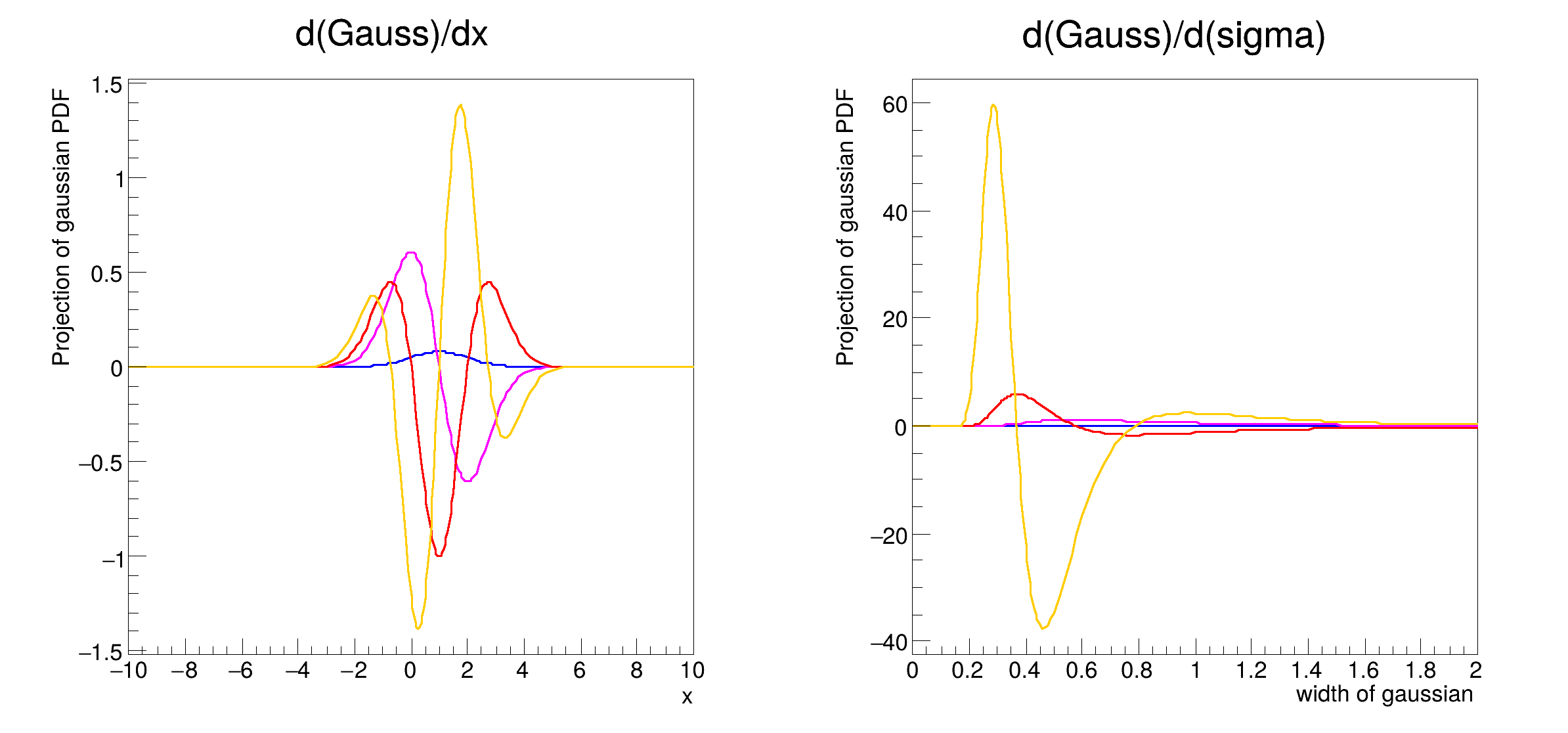ROOT   Reference Guide
Searching...
No Matches
rf111_derivatives.C File Reference

## Detailed DescriptionBasic functionality: numerical 1st,2nd and 3rd order derivatives w.r.t.

observables and parameters

pdf = gauss(x,m,s)
Double_t x[n]
Definition legend1.C:17
TMarker m
Definition textangle.C:8#include "RooRealVar.h"
#include "RooDataSet.h"
#include "RooGaussian.h"
#include "TCanvas.h"
#include "TAxis.h"
#include "RooPlot.h"
using namespace RooFit;
{
// S e t u p m o d e l
// ---------------------
// Declare variables x,mean,sigma with associated name, title, initial value and allowed range
RooRealVar x("x", "x", -10, 10);
RooRealVar mean("mean", "mean of gaussian", 1, -10, 10);
RooRealVar sigma("sigma", "width of gaussian", 1, 0.1, 10);
// Build gaussian pdf in terms of x,mean and sigma
RooGaussian gauss("gauss", "gaussian PDF", x, mean, sigma);
// C r e a t e a n d p l o t d e r i v a t i v e s w . r . t . x
// ----------------------------------------------------------------------
// Derivative of normalized gauss(x) w.r.t. observable x
RooAbsReal *dgdx = gauss.derivative(x, 1);
// Second and third derivative of normalized gauss(x) w.r.t. observable x
RooAbsReal *d2gdx2 = gauss.derivative(x, 2);
RooAbsReal *d3gdx3 = gauss.derivative(x, 3);
// Construct plot frame in 'x'
RooPlot *xframe = x.frame(Title("d(Gauss)/dx"));
// Plot gauss in frame (i.e. in x)
gauss.plotOn(xframe);
// Plot derivatives in same frame
dgdx->plotOn(xframe, LineColor(kMagenta));
d2gdx2->plotOn(xframe, LineColor(kRed));
d3gdx3->plotOn(xframe, LineColor(kOrange));
// C r e a t e a n d p l o t d e r i v a t i v e s w . r . t . s i g m a
// ------------------------------------------------------------------------------
// Derivative of normalized gauss(x) w.r.t. parameter sigma
RooAbsReal *dgds = gauss.derivative(sigma, 1);
// Second and third derivative of normalized gauss(x) w.r.t. parameter sigma
RooAbsReal *d2gds2 = gauss.derivative(sigma, 2);
RooAbsReal *d3gds3 = gauss.derivative(sigma, 3);
// Construct plot frame in 'sigma'
RooPlot *sframe = sigma.frame(Title("d(Gauss)/d(sigma)"), Range(0., 2.));
// Plot gauss in frame (i.e. in x)
gauss.plotOn(sframe);
// Plot derivatives in same frame
dgds->plotOn(sframe, LineColor(kMagenta));
d2gds2->plotOn(sframe, LineColor(kRed));
d3gds3->plotOn(sframe, LineColor(kOrange));
// Draw all frames on a canvas
TCanvas *c = new TCanvas("rf111_derivatives", "rf111_derivatives", 800, 400);
c->Divide(2);
c->cd(1);
xframe->GetYaxis()->SetTitleOffset(1.6);
xframe->Draw();
c->cd(2);
sframe->GetYaxis()->SetTitleOffset(1.6);
sframe->Draw();
}
#define c(i)
Definition RSha256.hxx:101
@ kRed
Definition Rtypes.h:66
@ kOrange
Definition Rtypes.h:67
@ kMagenta
Definition Rtypes.h:66
Abstract base class for objects that represent a real value and implements functionality common to al...
Definition RooAbsReal.h:59
RooDerivative * derivative(RooRealVar &obs, Int_t order=1, double eps=0.001)
Return function representing first, second or third order derivative of this function.
virtual RooPlot * plotOn(RooPlot *frame, const RooCmdArg &arg1={}, const RooCmdArg &arg2={}, const RooCmdArg &arg3={}, const RooCmdArg &arg4={}, const RooCmdArg &arg5={}, const RooCmdArg &arg6={}, const RooCmdArg &arg7={}, const RooCmdArg &arg8={}, const RooCmdArg &arg9={}, const RooCmdArg &arg10={}) const
Plot (project) PDF on specified frame.
Plain Gaussian p.d.f.
Definition RooGaussian.h:24
Plot frame and a container for graphics objects within that frame.
Definition RooPlot.h:43
static RooPlot * frame(const RooAbsRealLValue &var, double xmin, double xmax, Int_t nBins)
Create a new frame for a given variable in x.
Definition RooPlot.cxx:237
TAxis * GetYaxis() const
Definition RooPlot.cxx:1276
void Draw(Option_t *options=nullptr) override
Draw this plot and all of the elements it contains.
Definition RooPlot.cxx:649
Variable that can be changed from the outside.
Definition RooRealVar.h:37
virtual void SetTitleOffset(Float_t offset=1)
Set distance between the axis and the axis title.
Definition TAttAxis.cxx:298
The Canvas class.
Definition TCanvas.h:23
const Double_t sigma
The namespace RooFit contains mostly switches that change the behaviour of functions of PDFs (or othe...
Definition JSONIO.h:26
Ta Range(0, 0, 1, 1)
[#1] INFO:NumericIntegration -- RooRealIntegral::init(gauss_Int[sigma]) using numeric integrator RooIntegrator1D to calculate Int(sigma)
Date
July 2008

Definition in file rf111_derivatives.C.